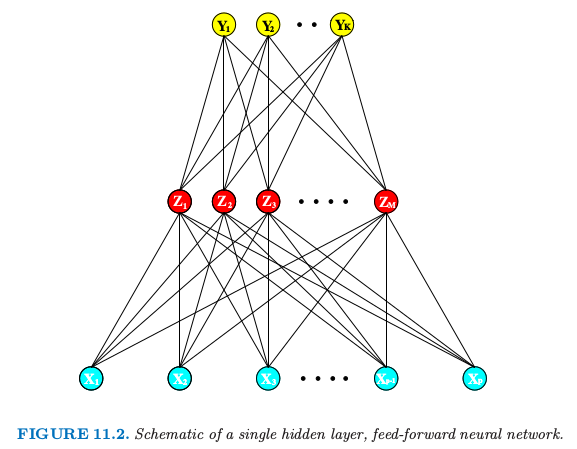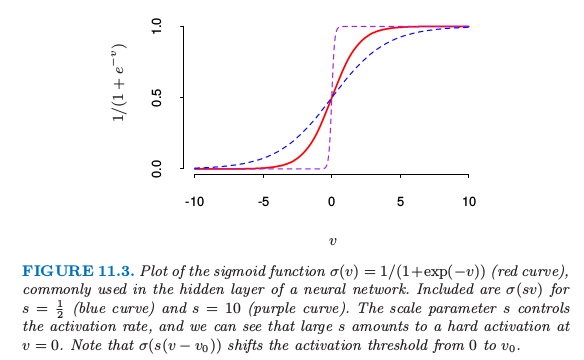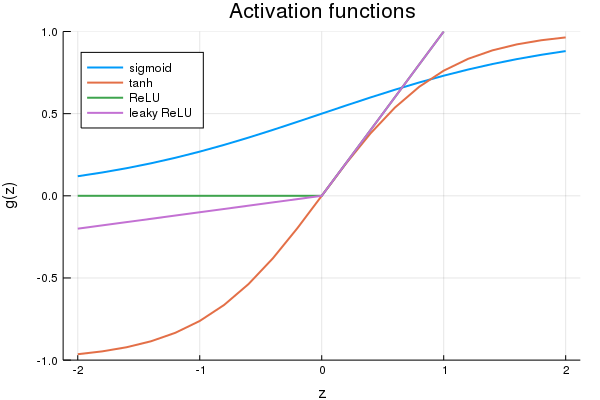11.3 神经网络¶weiya 注：其他形式的激活函数

• tanh: $g(z) = \frac{e^z-e^{-z}}{e^z+e^{-z}}$
• rectified linear unit (ReLU): $g(z) = z_+$
• leaky rectified linear unit: $g_\alpha(z)=z_+-\alpha z_-$weiya 注：Ex. 11.1

Ex. 11.1 讨论了单层回归（分类）神经网络与 PPR 模型的等价性．该题已解决，欢迎讨论，详见 Issue 171: Ex. 11.1